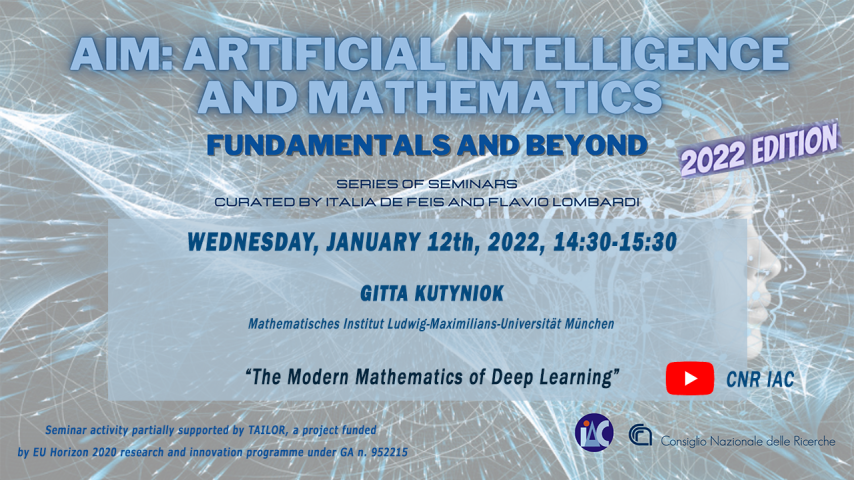# Gitta Kutyniok - The Modern Mathematics of Deep LearningDespite the outstanding success of deep neural networks in real-world applications, ranging from science to public life, most of the related research is empirically driven and a comprehensive mathematical foundation is still missing. At the same time, these methods have already shown their impressive potential in mathematical research areas such as imaging sciences, inverse problems, or numerical analysis of partial differential equations, sometimes by far outperforming classical mathematical approaches for particular problem classes. The goal of this lecture is to first provide an introduction into this new vibrant research area. We will then survey recent advances in two directions, namely the development of a mathematical foundation of deep learning and the introduction of novel deep learning-based approaches to solve inverse problems and partial differential equations.

Data inizio